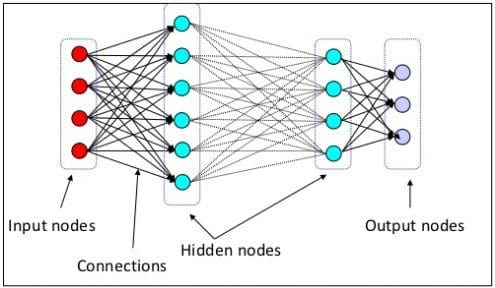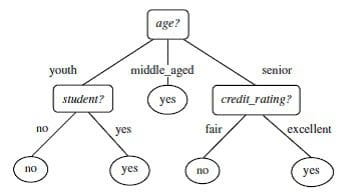# Great Collection of Minimal and Clean Implementations of Machine Learning Algorithms

Interested in learning machine learning algorithms by implementing them from scratch? Need a good set of examples to work from? Check out this post with links to minimal and clean implementations of various algorithms.

Want to implement machine learning algorithms from scratch?

A recent KDnuggets poll asked "Which methods/algorithms you used in the past 12 months for an actual Data Science-related application?" with results found here. The results are analyzed by industry employment sector and region, but the main take away for the uninitiated is that there are a wide array of algorithms covered.And let's be clear: this is not a complete representation of available machine learning algorithms, but rather a subset of the most-used algorithms (as per our readers). There are lots of machine learning algorithms in existence today. But with so many machine learning libraries, projects, programs, and other projects out there, covering so many of these algorithms, for a variety of programming languages and different platforms and environments, why on Earth would you ever want to implement an algorithm from scratch?

Sebastian Raschka, noted machine learning enthusiast, "data scientist" (his quotes, note mine), PhD candidate, and author of Python Machine Learning has this to say about implementing machine learning algorithms from scratch:

There are several different reasons why implementing algorithms from scratch can be useful:

1. it can help us to understand the inner works of an algorithm
2. we could try to implement an algorithm more efficiently
3. we can add new features to an algorithm or experiment with different variations of the core idea
4. we circumvent licensing issues (e.g., Linux vs. Unix) or platform restrictions
5. we want to invent new algorithms or implement algorithms no one has implemented/shared yet
6. we are not satisfied with the API and/or we want to integrate it more "naturally" into an existing software library

So, let's say you have a reason to implement machine learning algorithms from scratch. Or, alternatively, let's say you simply want a better understanding of how machine learning algorithms work (#1), or want to add new features to an algorithm (#3), or something else entirely. Locating a collection of independently-implemented algorithms, preferably from the same author in order to make the code easier to follow from one algorithm to the next, and (especially) implemented in minimal, clean code, can be a challenging undertaking.

Enter the MLAlgorithms Github Repository, by rushter, described via the repo README as:

A collection of minimal and clean implementations of machine learning algorithms.

And that's actually a very good description of the included code. As someone who has both implemented numerous machine learning algorithms from scratch -- having had to look through textbooks, papers, blog post, and code for direction -- and investigated algorithm code in order to understand how particular implementations worked in order to make modifications, I can say from experience that the code in this code is quite easy to follow.

This project is targeting people who want to learn internals of ml algorithms or implement them from scratch. The code is much easier to follow than the optimized libraries and easier to play with. All algorithms are implemented in Python, using numpy, scipy and autograd.

So, important points: algorithms are not optimized, so probably not good for production; algorithms implemented in Python with only a few common scientific computing libraries. As long as you are good with these points, and are interested in learning how to implement algorithms or simply understand them better, this code is for you.

As of writing, rushter has implemented algorithms of various task types, including clustering, classification, regression, dimensionality reduction, and a variety of deep neural network algorithms. In an attempt to help you coordinate learning by implementing, what follows is a partial list of implemented algorithm links as well as some supporting material. Note that all credit for these implemented algorithms goes to the repo's owner, rushter, whose Github profile indicates that he is available for hire. I would suspect this repo would be a well-received resume.

Deep Learning (MLP, CNN, RNN, LSTM)Start here: 7 Steps to Understanding Deep Learning

Go from vague understanding of deep neural networks to knowledgeable practitioner in 7 steps!

The code: rushter's neuralnet module

Linear Regression/Logistic RegressionStart here: Linear Regression, Least Squares & Matrix Multiplication: A Concise Technical Overview

Linear regression is a simple algebraic tool which attempts to find the “best” line fitting 2 or more attributes.

The code: rushter's linear_models.py

Random ForestsStart here: Random Forest: A Criminal Tutorial

Get an overview of Random Forest here, one of the most used algorithms by KDnuggets readers according to a recent poll.

The code: rushter's linear_models.py

Support Vector MachinesStart here: Support Vector Machines: A Concise Technical Overview

Support Vector Machines remain a popular and time-tested classification algorithm. This post provides a high-level concise technical overview of their functionality.

The code: rushter's svm.py, and rushter's kernels.py

K-nearest NeighborsStart here: Implementing Your Own k-Nearest Neighbour Algorithm Using Python

A detailed explanation of one of the most used machine learning algorithms, k-Nearest Neighbors.

The code: rushter's knn.py

Naive BayesStart here: Bayesian Machine Learning, Explained

Get a great introductory Bayesian machine learning explanation here, as well as suggestions where to go for further study.

The code: rushter's naive_bayes.py
K-Means ClusteringStart here: Comparing Clustering Techniques: A Concise Technical Overview

A wide array of clustering techniques are in use today. Given the widespread use of clustering in everyday data mining, this post provides a concise technical overview of 2 such exemplar techniques.

The code: rushter's kmeans.py

All implemented algorithms come with example code, which is helpful for understanding the APIs. An example is as follows:

The above algorithm implementations are a sample of what is included in the repo, so be sure to have a look at the following implementations as well:

If you have found these code examples helpful, I'm sure the author would be happy to hear from you.

Related: